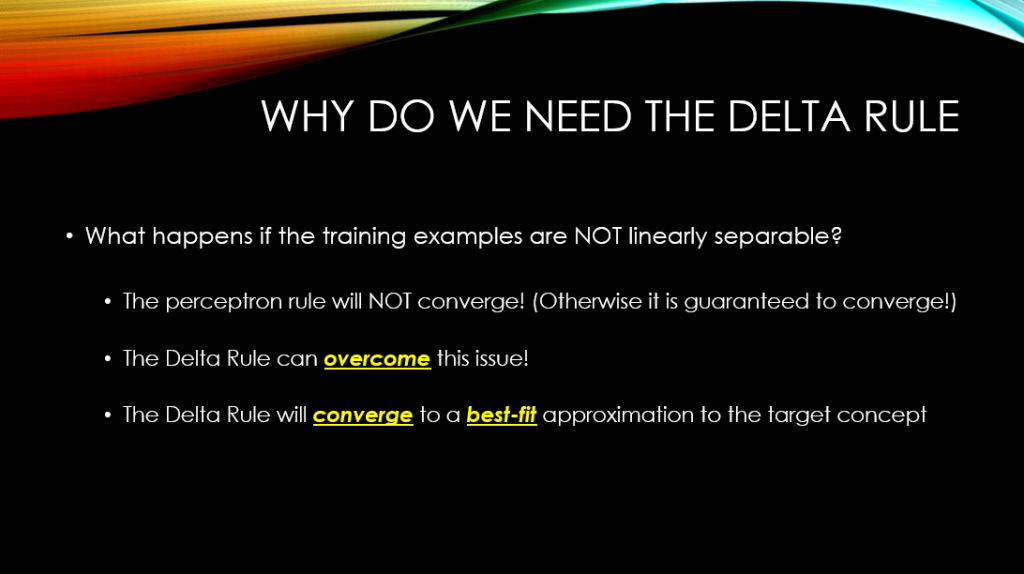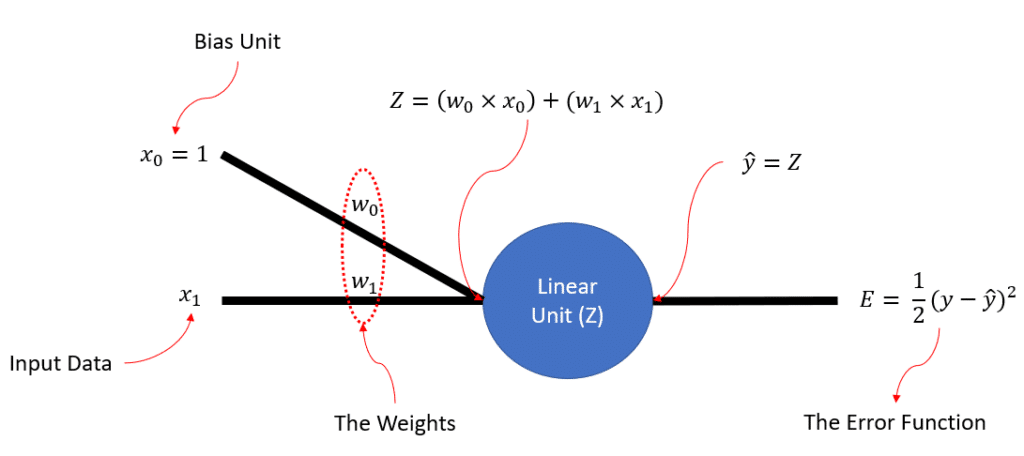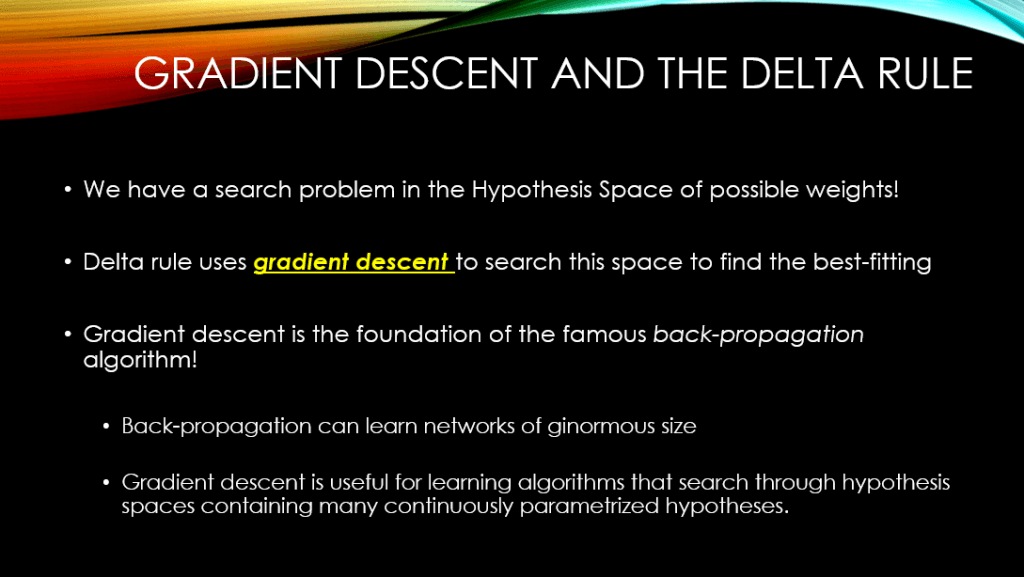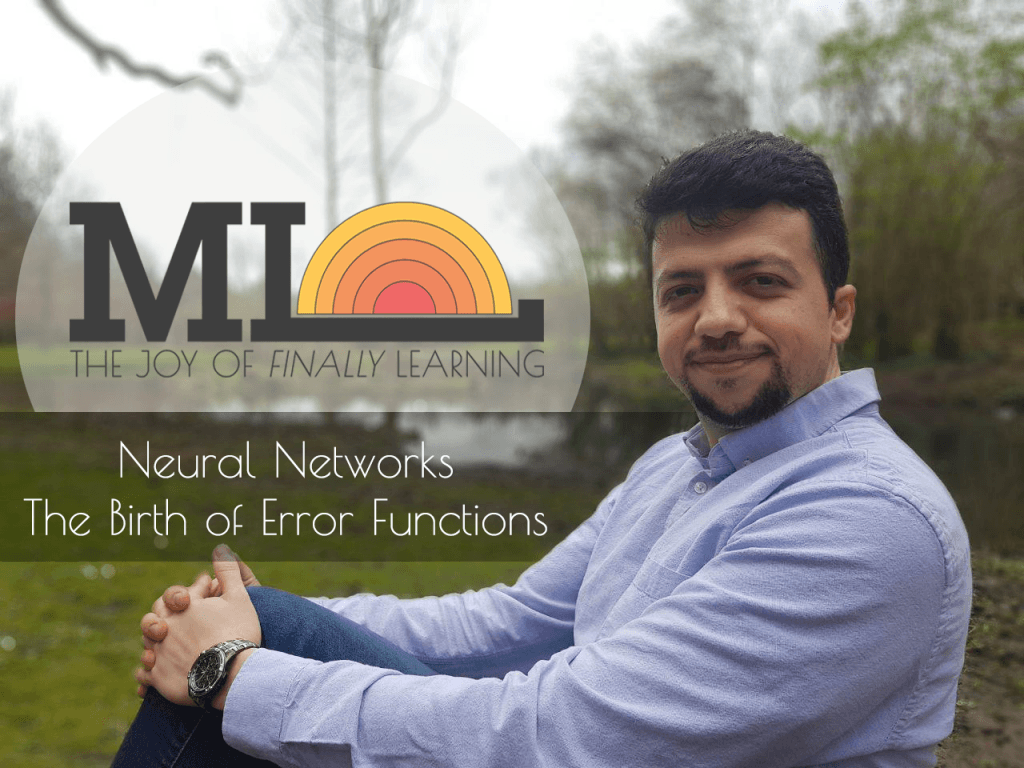## The Beauty that is the Delta Rule

In general, there are 2 main ways to train an Artificial Neural Network (ANN). In our previous post , I have told you about the popular perceptron rule that has been around for a long time. We also said that the perceptron training rule is guaranteed to converge if and only if the training examples are linearly separable. However, there are cases that the pereptron training rule would simply fail! More specifically, if the data are not linearly separable, the perceptron training rule will simply NOT converge! This is when the Delta rule comes to the rescue. The big picture of why the Delta rule is famous is displayed down below, pictorially:## The Delta Rule is as a Searcher

Let’s remind ourselves of the following fact:

When a machine learns, it means that it is searching in a hypothesis space, with the sole goal of finding a hypothesis that fits the training data the best.

As a gentle reminder, you can watch the video down below to refresh your memory on the very meaning of a hypothesis, the hypothesis space, and searching in a hypothesis space:

So consider the simple neural network down below. Let’s say we would like to build a binary classifier that would generate a value close to 1, if our data x belongs to the positive class, and 0, if it belongs to the negative class. So, we will have to learn a set of weights in our weight space, that would force this neural network to generate our desired outputs, given our input.So, you can say that the weight space is indeed your hypothesis space, where you have loads and loads of possible values for these weights. However, only a subset of these weights can turn your neural network into a successful model with a high degree of performance on your data.

The Delta Rule is an interesting mechanism for searching the hypothesis space. Actually, the Delta Rule uses one of the most, if not the most, popular search technique in the hypothesis space that is called Gradient Descent.

Using Gradient Descent, the Delta Rule strives to find the best-fitting model. In other words:

What are the weights that would make my neural network fit the training data the best, with the highest performance –> Least amount of error!

The Delta Rule, uses gradient descent as an optimization techniques, and tries different values for the weights in a neural network, and depending on how accurate the output of the network is (i.e., how close to the ground truth), it will make certain adjustments to certain weights (i.e., increase some and decrease the other). It will try to increase and decrease the weights in a way that the error of the output would go down, during training. So, in summary:Just as a side note, gradient descent is the very foundation of the back-propagation algorithm that helps us learn neural networks of ginormous size (i.e., Deep Learning).

## Gradient Descent and the Delta Rule

Let’s consider the neural network that we talked about earlier in this post, where we have 1-dimensional input data, and the activation function in the output neuron is linear. This means that the input and the output of this neuron is identical, as if the neuron makes no changes to its input. This network is slightly different from the popular perceptron that we discussed in our previous post. The difference is simply in the activation functions in both of them:

1. The perceptron, has a step function in its output neuron that outputs only 2 values, namely, -1 and +1. This is an architecture designed for a binary classification dataset. Moreover, we encourage the network to learn the weights that would make the network produce the correct +1 and -1 for the + and – examples in our training set.
2. Here, however, the activation function is linear. This means that the output of this neural network can be any real number. This is a nice architecture for a regression problem, where we would like the network to produce a real value, predicting a certain metric, measurement, etc. For example, the price of the oil in the next week, or the number of sold cars by tomorrow afternoon.

As a result, the output of the perceptron has changed from:to the following:Finally, in order for our Delta Rule to work, we need to have a measure to quantify the performance of our network. Meaning, how far away are the outputs from the ground truth. This measure will be our error function. So, for every inputand the choice of weight vector (from the hypothesis space), how far is our output from the ground truth.

One common error function that can be used here, is the Sum Square Error (SSE) error function:Where:

•: The ground truth for the training example•: The output of the linear perceptron for the training example•: The training set

So, if you think about it, this error function measures the difference between the generated output and the ground truth for every exampleacross the whole training set. Note that the error is a function of our weight vector. The Delta rule searches for these weight vectors and uses them to generate the output for a given training example. Then by measuring the error, it would update the previously chosen weights to new values in a way that the output for the subsequent training examples would get closer to the ground truth. So, the more the training goes on the better weights would the Delta rule find, and the error for those weights would become less and less. Until eventually, the network has converged and we say that the model has been trained.

You might wonder why we have thebit, or why this particular error function is chosen. I am not going to answer this question in this post as this has something to do with the Bayes’ rule and it is beyond our current post. Having said that I will leave you with a claim:

From a Bayesian perspective, under certain conditions, it can be shown that the hypothesis that minimizes this particular error function, is also the most probable hypothesis (i.e., weights) given the training data.

In plain English:

The hypothesis that minimizes this particular error function, is the one that maximizes the probability of observing values in the output of our model, as close as possible to the ground truth.

Side note: In case you would like to see the actual derivation of this error function from a Bayesian perspective, when dealing with a neural network with a linear output, I would recommend our course at MLDawn, down below:## Conclusions

Today we have learned that the Delta rule is a search method in the hypothesis space that uses gradient descent as an optimization technique. We also learned that the perceptron rule would not converge to any solution if the training data are not linearly separable, and that is when the Delta rule comes to rescue as it would converge to the best fit model.

In the next post, we will have a nice visualization of the Sum Square Error (SSE) function surface in 2 dimensions, where each dimension demonstrates 1 weight in our neural network (i.e., we have 2 weights in our neural network). We will actually see that there is indeed a pair of weights that will touch the error surface in its minimum, meaning that if the model finds those weights in its search through the hypothesis space, then the model has converged and it has been trained.

Until then, on behalf of MLDawn,

Take care 😉